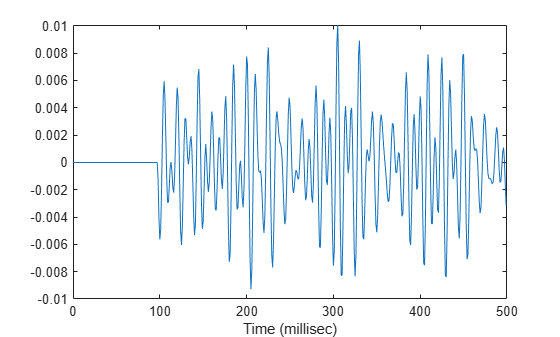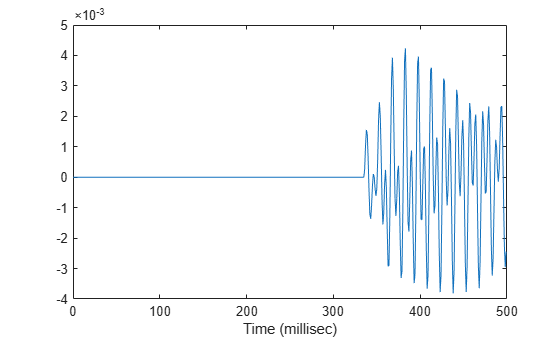# step

System object: phased.MultipathChannel
Package: phased

Propagate signal through multipath sound channel

## Syntax

``propsig = step(propagator,sig,pathmat,dop,aloss)``

## Description

Note

Instead of using the `step` method to perform the operation defined by the System object™, you can call the object with arguments, as if it were a function. For example, ```y = step(obj,x)``` and `y = obj(x)` perform equivalent operations.

example

````propsig = step(propagator,sig,pathmat,dop,aloss)` returns a signal, `propsig`, propagated through a multipath channel. `sig` is the input signal to the channel. The `pathmat` matrix contains the path time delay, the total reflection coefficient, and the spreading loss. `dop` specifies the Doppler factor and `aloss` specifies the frequency-dependent absorption loss. The matrix can describe one-way or two-way propagation from the signal source position to the signal destination position.When you use this method for one-way propagation, the source refers to the origin of the signal and the destination refers to the receiver. You can use one-way propagation modeling to model passive sonar and underwater communications.When you use this method for two-way propagation, the destination refers to the reflecting target, not the sonar receiver. A two-way path consists of a two identical one-way paths from source to target and back to receiver (collocated with the source). You can use two-way propagation to model active sonar systems.```

Note

The object performs an initialization the first time the object is executed. This initialization locks nontunable properties and input specifications, such as dimensions, complexity, and data type of the input data. If you change a nontunable property or an input specification, the System object issues an error. To change nontunable properties or inputs, you must first call the `release` method to unlock the object.

Note

Instead of using the `step` method to perform the operation defined by the System object, you can call the object with arguments, as if it were a function. For example, ```y = step(obj,x)``` and `y = obj(x)` perform equivalent operations.

## Input Arguments

expand all

Multipath channel propagator, specified as a `phased.MultipathChannel` System object.

Example: `phased.MultipathChannel`

Channel input signal, specified as a complex-valued M-by-N matrix. M is the number of samples in the signal and N is the number of paths.

Data Types: `double`
Complex Number Support: Yes

Propagation paths matrix, specified as a real-valued 3-by-N matrix. N is the number of paths in the channel. Each column represents a path. The matrix rows represent:

RowData
1Propagation delays for each path. Units are in seconds.
2Total reflection coefficient for each path. Units are dimensionless
3Spreading loss for each path. Units are in dB.

Except for the direct path, paths consist of alternating surface and bottom reflections. The losses for multiple reflections at the boundaries are multiplied. When you use `phased.IsoSpeedUnderwaterPaths` to create a path matrix, some of the columns can contain `NaN` values. `phased.MultipathChannel` ignores these paths.

Data Types: `double`

Doppler factor, specified as a real-valued N-by-1 row vector where N is the number of paths. The Doppler factor multiplies the transmitted frequency to produce the Doppler-shifted frequency for each path. The factor also defines the time contraction or dilation of a signal. Units are dimensionless.

Data Types: `double`

Frequency-dependent absorption loss, specified as a real-valued K-by-N+1 matrix. K is the number of frequencies and N is the number of paths. The first column of `aloss` contains the absorption-loss frequencies in Hz. The remaining columns contain the absorption losses for the corresponding frequency. Units are in dB.

Data Types: `double`

## Output Arguments

expand all

Channel output signal, returned as a complex-valued M-by-N matrix. M is the number of samples in the signal and N is the number of paths. The output is the signal propagated through the channel. `propsig` has the same dimensions as the input signal, `sig`.

## Examples

expand all

Create a five-path underwater sound channel and compute the propagation path matrix, the Doppler factor, and the absorption loss. Assume that the source is stationary and the receiver is moving along the x-axis toward the source at 20 km/h. Assume the default one-way propagation.

Create the channel and specify the source and receiver locations and velocities.

```numpaths = 5; channel = phased.IsoSpeedUnderwaterPaths('ChannelDepth',200,'BottomLoss',10, ... 'NumPathsSource','Property','NumPaths',numpaths); tstep = 1; srcpos = [0;0;-160]; rcvpos = [100;0;-50]; speed = -20*1000/3600; srcvel = [0;0;0]; rcvvel = [speed;0;0];```

Compute the path matrix, Doppler factor, and losses.

`[pathmat,dop,absloss] = channel(srcpos,rcvpos,srcvel,rcvvel,tstep);`

Create 500 samples of a 100 Hz signal. Assume all the paths have the same signal. Propagate the signals to the receiver.

```fs = 1e3; nsamp = 500; propagator = phased.MultipathChannel('OperatingFrequency',10e3,'SampleRate',fs); t = [0:(nsamp-1)]'/fs; sig0 = sin(2*pi*100*t); sig = repmat(sig0,1,numpaths); propsig = propagator(sig,pathmat,dop,absloss);```

Plot the real part of the coherent sum of the propagated signals.

```plot(t*1000,real(sum(propsig,2))) xlabel('Time (millisec)')```Create a seven-path underwater sound channel and display the propagation path matrix. Assume that the source is stationary and that the receiver is moving along the x-axis toward the source at 20 km/h. Assume two-way propagation.

```speed = -20*1000/3600; numpaths = 7; csound = 1515.0; channel = phased.IsoSpeedUnderwaterPaths('ChannelDepth',200, ... 'PropagationSpeed',csound,'BottomLoss',10,'NumPathsSource','Property', ... 'NumPaths',numpaths,'TwoWayPropagation',true); tstep = 1; srcpos = [0;0;-160]; tgtpos = [500;0;-50]; srcvel = [0;0;0]; tgtvel = [speed;0;0];```

Obtain the path matrix, Doppler factor, loss, and target reflection and transmit angles.

`[pathmat,dop,aloss,tgtangs,srcangs] = channel(srcpos,tgtpos,srcvel,tgtvel,tstep);`

Create a 100 Hz signal with 500 samples. Assume that all the paths have the same signal but with different amplitudes. Then, propagate the signals to the target and back. You can use the angle information to calculate any angular dependence of the source and target responses. Each channel can have a different amplitude. This example uses a simple cosine model.

```fs = 1e3; nsamp = 500; propagator = phased.MultipathChannel('OperatingFrequency',10e3,'SampleRate',fs); t = [0:(nsamp-1)]'/fs; ampsrc = cosd(srcangs(2,:)); amptgt = cosd(tgtangs(2,:)); sig0 = sin(2*pi*100*t); sig = repmat(sig0,1,numpaths); amptotal = ampsrc.^2.*amptgt; sig = bsxfun(@times,amptotal,sig);```

Because of the finite propagation delay, the first call to the propagator does not return the signal. Call `propagator` twice to obtain the returned signal.

```propsig = propagator(sig,pathmat,dop,aloss); propsig = propagator(sig,pathmat,dop,aloss);```

Plot the real part of the coherent sum of the propagated signals. Compute the round trip time.

```rng = rangeangle(srcpos,tgtpos); tr = rng/csound; plot((t+tr)*1000,real(sum(propsig,2))) xlabel('Time (millisec)')```Create an underwater sound channel and plot the combined received signal. Automatically find the number of paths. Assume that the source is stationary and that the receiver is moving along the x-axis toward the source at 20 km/h. Assume the default one-way propagation.

```speed = -20*1000/3600; channel = phased.IsoSpeedUnderwaterPaths('ChannelDepth',200,'BottomLoss',5, ... 'NumPathsSource','Auto','CoherenceTime',5); tstep = 1; srcpos = [0;0;-160]; rcvpos = [500;0;-50]; srcvel = [0;0;0]; rcvvel = [speed;0;0];```

Compute the path matrix, Doppler factor, and losses. The propagator outputs 51 paths output but some paths can contain `Nan` values.

`[pathmat,dop,absloss,rcvangs,srcangs] = channel(srcpos,rcvpos,srcvel,rcvvel,tstep);`

Create of a 100 Hz signal with 500 samples. Assume that all the paths have the same signal. Use a `phased.MultipathChannel` System object™ to propagate the signals to the receiver. `phased.MultipathChannel` accepts as input all paths produced by `phased.IsoSpeedUnderwaterPaths` but ignores paths that have `NaN` values.

```fs = 1e3; nsamp = 500; propagator = phased.MultipathChannel('OperatingFrequency',10e3,'SampleRate',fs); t = [0:(nsamp-1)]'/fs; sig0 = sin(2*pi*100*t); numpaths = size(pathmat,2); sig = repmat(sig0,1,numpaths); propsig = propagator(sig,pathmat,dop,absloss);```

Plot the real part of the coherent sum of the propagated signals.

```plot(t*1000,real(sum(propsig,2))) xlabel('Time (millisec)')```## Version History

Introduced in R2017a